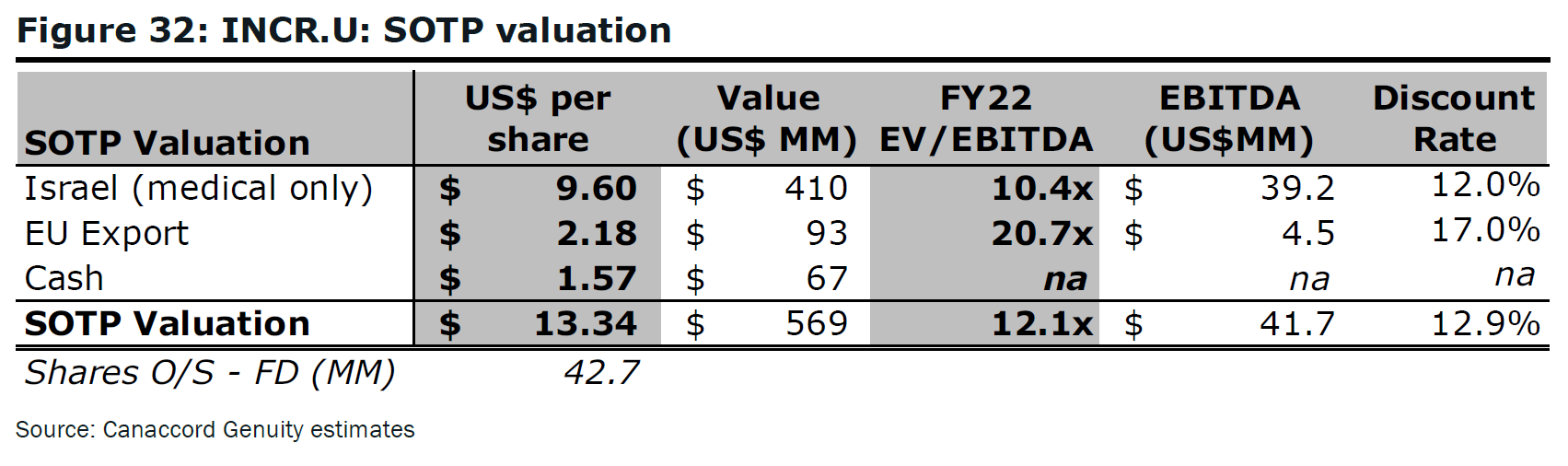# Fight Finance

#### CoursesTagsRandomAllRecentScores

To value a business's assets, the free cash flow of the firm (FCFF, also called CFFA) needs to be calculated. This requires figures from the firm's income statement and balance sheet. For what figures is the income statement needed? Note that the income statement is sometimes also called the profit and loss, P&L, or statement of financial performance.

If a firm makes a profit and pays no dividends, which of the firm’s accounts will increase?

Where can a publicly listed firm's book value of equity be found? It can be sourced from the company's:

Where can a private firm's market value of equity be found? It can be sourced from the company's:

Which of the following formulas for 'contributed equity' from the balance sheet is correct? Assume that now is time 1 and last year is time 0. Assume that book equity consists of contributed equity, retained profits and reserves only (BookEquity = ContributedEquity + RetainedProfits + Reserves).

Here is a table from Canaccord's 'sum of the parts' valuation of INCR.Note that the firm INCR is unlevered (interest bearing debt = 0).

The third column from the left is labelled 'Value (US\$ MM)'. For the 'Israel' and 'EU Export' opportunities, these values are most likely to be: